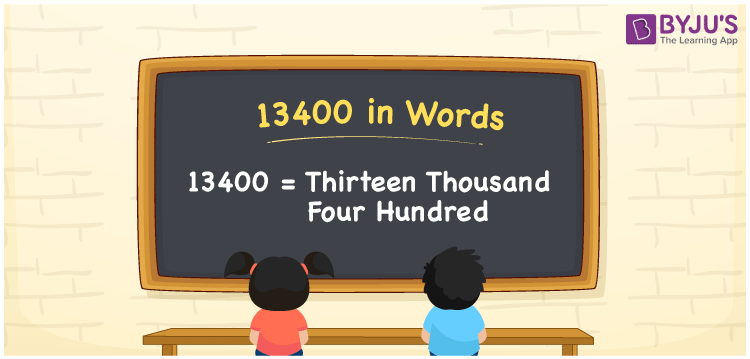# 13400 in Words

13400 in words is written as Thirteen thousand four hundred. In both the International System of Numerals and the Indian System of Numerals, 13400 in words can be written as Thirteen thousand four hundred. The number 13400 is a Cardinal Number as it represents some quantity. For example, “this mobile phone costs 13400 rupees”.

 13400 in words Thirteen thousand four hundred Thirteen thousand four hundred in numerals 13400

## 13400 in English Words

13400 in English words is read as “Thirteen thousand four hundred.”## How to Write 13400 in Words?

To write 13400 in words, we shall use the place value chart. In the place value chart, put 1 in the ten thousands, 3 in the thousands, 4 in the hundreds, and 0 in the tens and the ones, respectively. Let us make a place value chart to write the number 13400 in words.

 Ten Thousands Thousands Hundreds Tens Ones 1 3 4 0 0

Thus, we can write the expanded form as

1 × Ten Thousand + 3 × Thousand + 4 × Hundred + 0 × Ten + 0 × One

= 1 × 10000 + 3 × 1000 + 4 × 100 + 0 × 10 + 0 × 1

= 10000 + 3000 + 400 + 0 + 0

= 13400

= Thirteen thousand four hundred.

13400 is a natural number between 13399 and 13401.

13400 in words – Thirteen thousand four hundred

• Is 13400 an odd number? – No
• Is 13400 an even number? – Yes
• Is 13400 a perfect square number? – No
• Is 13400 a perfect cube number? – No
• Is 13400 a prime number? – No
• Is 13400 a composite number? – Yes

## Frequently Asked Questions on 13400 in Words

Q1

### How to write 13400 in words?

13400 in words can be written as Thirteen thousand four hundred.
Q2

### How to write 13400 in words in the International and Indian System of Numerals?

In both, the system of numerals, 13400 in words, is written as Thirteen thousand four hundred.
Q3

### How to write 13400 in a place value chart?

In the place value chart, write 1 in the ten thousands, 3 in the thousands, 4 in the hundreds, and 0 in the tens and the ones, respectively.
Test your Knowledge on 13400 in Words